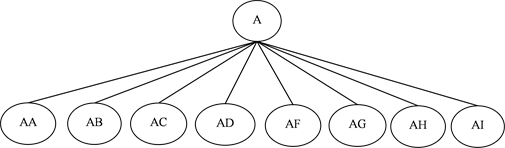# 服装企业层次时间序列销售预测协调探究Exploration of Hierarchical Time Series Sales Forecast of Apparel Enterprises

DOI: 10.12677/MM.2017.76061, PDF, HTML, XML, 下载: 1,044  浏览: 1,969

Abstract: Sales forecasting is the foundation of business decisions. As to the problem that there is disunity phenomenon among the process of hierarchical time series forecasting due to neglection of the connection between different hierarchies and the unicity of the sales forecast object, this paper aims to explore the hierarchical sales forecasting coordination methods. It compares four coordi-nation methods, “top-down” method, “bottom-up” method, and “top-down” method based on pre-dicted value proportion and method based on least square estimation and predicted value pro-portion proposed by this paper. Then, it uses actual apparel sales data to verify these methods. Results show that new methods perform better at forecasting accuracy than other two traditional methods on the basis of coordination of forecasting results. This could provide new train of thought for enterprises which have the demand of hierarchical sales forecasting.

1. 引言Figure 1. Structure of hierarchical time series

2. 整体算法及流程

1) 收集待预测服装销售历史数据；

2) 处理数据，将销售数据按照服装品类进行整理；

3) 对服装总销售额以及各服装品类销售额进行按季度的销售预测；

4) 分别运用由上至下，右下至上，以及按底层预测值比例改进的由上至下法和基于最小二乘思想及底层预测值比例的层次协调预测法，处理上一步得到的预测值；

5) 得到服装品类维度上下层统一的季度销售预测值，作为最终的预测结果。

2.1. 由上至下及由下至上方法

$x$ ，产品时间序列

${Y}_{x,t}$ ，序列x在t时刻的观测值

$\stackrel{^}{{Y}_{x,t}}$ ，序列x在t时刻的预测值

$\stackrel{˜}{{Y}_{x,t}}$ ，序列x在t时刻协调后的预测值

${Y}_{t}$ ，t时刻总序列观测值

$\stackrel{^}{{Y}_{t}}$ ，t时刻总序列预测值

$\stackrel{˜}{{Y}_{t}}$ ，t时刻总序列协调后预测值

${Y}_{i,t}$ ，t时刻层次i上所有序列的观测值序列集合

${Y}_{t}$ ，t时刻所有观测值序列的集合， ${Y}_{t}={\sum }_{i}{Y}_{i,t}$$i=1,2,\cdots ,k$

$\stackrel{^}{{Y}_{i,t}}$ ，t时刻层次i上所有序列的预测值序列集合

$\stackrel{^}{{Y}_{t}}$ ，t时刻所有预测值序列的集合， $\stackrel{^}{{Y}_{t}}={\sum }_{i}\stackrel{^}{{Y}_{i,t}}$$i=1,2,\cdots ,k$

$\stackrel{˜}{{Y}_{i,t}}$ ，t时刻层次i上所有协调后的预测值序列集合

$\stackrel{˜}{{Y}_{t}}$ ，t时刻所有协调后的预测值序列的集合， $\stackrel{˜}{{Y}_{t}}={\sum }_{i}\stackrel{˜}{{Y}_{i,t}}$$i=1,2,\cdots ,k$

$S$ ，以 $m\ast {m}_{K}$ 为结构的求和矩阵

$\stackrel{˜}{{Y}_{t}}=S\ast \stackrel{^}{{Y}_{t}}$

$S=\left[\begin{array}{lllll}1\hfill & 11\hfill & 11\hfill & 11\hfill & 1\hfill \\ 1\hfill & 00\hfill & 00\hfill & 00\hfill & 0\hfill \\ 0\hfill & 10\hfill & 00\hfill & 00\hfill & 0\hfill \\ 0\hfill & 01\hfill & 00\hfill & 00\hfill & 0\hfill \\ 0\hfill & 00\hfill & 10\hfill & 00\hfill & 0\hfill \\ 0\hfill & 00\hfill & 01\hfill & 00\hfill & 0\hfill \\ 0\hfill & 00\hfill & 00\hfill & 10\hfill & 0\hfill \\ 0\hfill & 00\hfill & 00\hfill & 01\hfill & 0\hfill \\ 0\hfill & 00\hfill & 00\hfill & 00\hfill & 1\hfill \end{array}\right]$结构为 ${m}_{K}\ast {m}_{K}$ 的单位矩阵

2.1.1. 由上至下

${P}_{x,t}=\frac{{\sum }_{t=1}^{n}\text{ }\text{ }{Y}_{x,t}}{n}/\frac{{\sum }_{t=1}^{n}\text{ }\text{ }{Y}_{t}}{n}$

$\stackrel{˜}{{Y}_{t}}={P}_{x,t}\ast S\ast \stackrel{^}{{Y}_{t}}$ (2)

$\left[\begin{array}{c}\begin{array}{c}{Y}_{A,t}\\ {Y}_{AA,t}\\ {Y}_{AB,t}\end{array}\\ {Y}_{AC,t}\\ {Y}_{AD,t}\\ {Y}_{AE,t}\\ {Y}_{AF,t}\\ {Y}_{AG,t}\\ {Y}_{AH,t}\end{array}\right]=\left[\begin{array}{c}\begin{array}{c}{P}_{AA,t}\\ {P}_{AB,t}\\ {P}_{AC,t}\end{array}\\ {P}_{AD,t}\\ {P}_{AE,t}\\ {P}_{AF,t}\\ {P}_{AG,t}\\ {P}_{AH,t}\end{array}\right]\ast \left[\begin{array}{lllll}1\hfill & 11\hfill & 11\hfill & 11\hfill & 1\hfill \\ 1\hfill & 00\hfill & 00\hfill & 00\hfill & 0\hfill \\ 0\hfill & 10\hfill & 00\hfill & 00\hfill & 0\hfill \\ 0\hfill & 01\hfill & 00\hfill & 00\hfill & 0\hfill \\ 0\hfill & 00\hfill & 10\hfill & 00\hfill & 0\hfill \\ 0\hfill & 00\hfill & 01\hfill & 00\hfill & 0\hfill \\ 0\hfill & 00\hfill & 00\hfill & 10\hfill & 0\hfill \\ 0\hfill & 00\hfill & 00\hfill & 01\hfill & 0\hfill \\ 0\hfill & 00\hfill & 00\hfill & 00\hfill & 1\hfill \end{array}\right]\ast \left[\stackrel{^}{{Y}_{t}}\right]$

2.1.2. 由下至上

$\stackrel{˜}{{Y}_{t}}=S\ast \stackrel{^}{{Y}_{K,t}}$ (3)

$\left[\begin{array}{c}\begin{array}{c}{Y}_{A,t}\\ {Y}_{AA,t}\\ {Y}_{AB,t}\end{array}\\ {Y}_{AC,t}\\ {Y}_{AD,t}\\ {Y}_{AE,t}\\ {Y}_{AF,t}\\ {Y}_{AG,t}\\ {Y}_{AH,t}\end{array}\right]=\left[\begin{array}{lllll}1\hfill & 11\hfill & 11\hfill & 11\hfill & 1\hfill \\ 1\hfill & 00\hfill & 00\hfill & 00\hfill & 0\hfill \\ 0\hfill & 10\hfill & 00\hfill & 00\hfill & 0\hfill \\ 0\hfill & 01\hfill & 00\hfill & 00\hfill & 0\hfill \\ 0\hfill & 00\hfill & 10\hfill & 00\hfill & 0\hfill \\ 0\hfill & 00\hfill & 01\hfill & 00\hfill & 0\hfill \\ 0\hfill & 00\hfill & 00\hfill & 10\hfill & 0\hfill \\ 0\hfill & 00\hfill & 00\hfill & 01\hfill & 0\hfill \\ 0\hfill & 00\hfill & 00\hfill & 00\hfill & 1\hfill \end{array}\right]\ast \left[\begin{array}{c}\begin{array}{c}{Y}_{AA,t}\\ {Y}_{AB,t}\\ {Y}_{AC,t}\end{array}\\ {Y}_{AD,t}\\ {Y}_{AE,t}\\ {Y}_{AF,t}\\ {Y}_{AG,t}\\ {Y}_{AH,t}\end{array}\right]$

3. 层次时间序列预测新方法

3.1. 基于底层预测值比例的由上至下法

${{P}^{\prime }}_{xt}=\stackrel{^}{{Y}_{x,t}}/\underset{i}{\sum }\text{ }\text{ }\stackrel{^}{{Y}_{x,t}}$

$\stackrel{˜}{{Y}_{t}}={{P}^{\prime }}_{x,t}\ast S\ast \stackrel{^}{{Y}_{1,t}}$ (4)

3.2. 基于底层预测值比例及最小二乘法的层次预测协调法

$\left\{\begin{array}{l}\mathrm{min}f={\sum }_{i=0}^{n}\text{ }\text{ }{{P}^{\prime }}_{xt}{\left(\frac{\stackrel{^}{{Y}_{i,t}}-\stackrel{˜}{{Y}_{i,t}}}{\stackrel{^}{{Y}_{i,t}}}\right)}^{2}\\ \text{s}\text{.t}\text{.}\text{\hspace{0.17em}}\text{\hspace{0.17em}}\stackrel{˜}{{Y}_{t}}={\sum }_{i=1}^{n}\text{ }\stackrel{˜}{{Y}_{i,t}}\end{array}$ (5)

$\stackrel{˜}{{Y}_{i,t}}=\stackrel{^}{{Y}_{i,t}}-\frac{\frac{{\stackrel{^}{{Y}_{i,t}}}^{2}}{{p}_{i}}}{{\sum }_{i=0}^{n}\frac{{\stackrel{^}{{Y}_{i,t}}}^{2}}{{p}_{i}}}\left({\sum }_{i=0}^{n}\text{ }\stackrel{^}{{Y}_{i,t}}-\stackrel{^}{{Y}_{t}}\right)=\stackrel{^}{{Y}_{i,t}}-\frac{\frac{{\stackrel{^}{{Y}_{i,t}}}^{2}}{{p}_{i}}}{{\sum }_{i=0}^{n}\frac{{\stackrel{^}{{Y}_{i,t}}}^{2}}{{p}_{i}}}\Delta$ (6)

$\stackrel{˜}{{Y}_{t}}=\stackrel{^}{{Y}_{t}}+\frac{{\stackrel{^}{{Y}_{t}}}^{2}}{{\sum }_{i=0}^{n}\text{ }{\stackrel{^}{{Y}_{i,t}}}^{2}}\left({\sum }_{i=0}^{n}\text{ }\stackrel{^}{{Y}_{i,t}}-\stackrel{^}{{Y}_{t}}\right)=\stackrel{^}{{Y}_{t}}+\frac{{\stackrel{^}{{Y}_{t}}}^{2}}{{\sum }_{i=0}^{n}\text{ }{\stackrel{^}{{Y}_{i,t}}}^{2}}\Delta$ (7)

4. 实证研究Table 1. Direct forecasting value of apparel categories and gross salesTable 2. Coordinated forecasting values of apparel categories and gross sales

5. 结论

  罗毅初, 冯天瑞, 李达凯, 等. 基于加和比例分配算法的中长期电量预测改进方法[J]. 电力需求侧管理, 2014, 16(4): 11-15.  康重庆, 牟涛, 夏清. 电力系统多级负荷预测及其协调问题(一)研究框架[J]. 电力系统自动化, 2008, 32(7): 34- 38.  Stone, R., Champernowne, D.G. and Meade, J.E. (1942) The Precision of National Income Estimates. Review of Economic Studies, 9, 111. https://doi.org/10.2307/2967664  Wrighton, N. and Buckingham, C.D. (1954) Linear Aggregation of Eco-nomic Relations. North-Holland Publishing Company, New York.  Hyndman, R.J., Ahmed, R.A., Athanasopoulos, G., et al. (2011) Optimal combination forecasts for hierarchical time series[J]. Computational Statistics & Data Analysis, 55, 2579-2589. https://doi.org/10.1016/j.csda.2011.03.006  Hyndman, R.J., Lee, A.J. and Wang, E. (2015) Fast Computation of Reconciled Forecasts for Hierarchical and Grouped Time Series. Monash Econometrics & Business Statistics Working Papers, 97, 16-32.  黄红选, 韩继业. 数学规划[M]. 北京: 清华大学出版社, 2006.